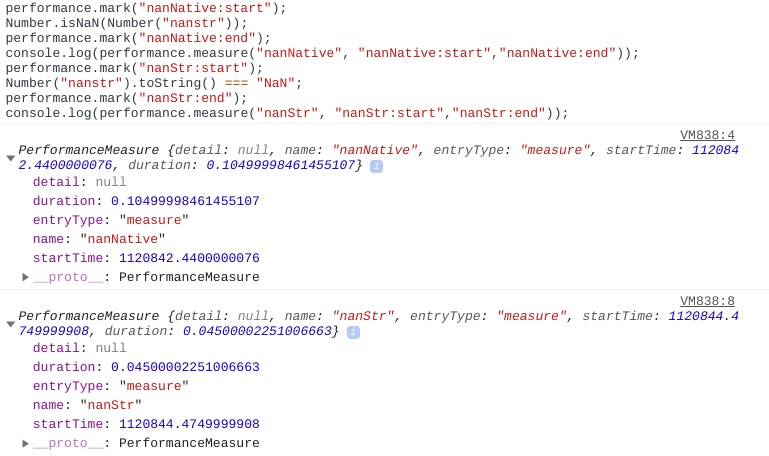Ask coding questions

← Back to all posts
##### isNan vs toString and check if "NaN"

I was wondering, why instead of doing this to check for NaN:

``Number.isNaN(Number("nanThing"));``

we don't do this:

``Number("nanThing").toString() === "NaN";``

I know that `NaN !== NaN`, but these two ways of checking if a number is `NaN` both work.

I did some performance tests (chrome 32-bit ver 77) to see if the `toString` method is slower:

``````performance.mark("nanNative:start");
Number.isNaN(Number("nanstr"));
performance.mark("nanNative:end");
console.log(performance.measure("nanNative", "nanNative:start","nanNative:end"));

performance.mark("nanStr:start");
Number("nanstr").toString() === "NaN";
performance.mark("nanStr:end");
console.log(performance.measure("nanStr", "nanStr:start","nanStr:end"));``````On the contrary, the `isNaN` is a bit slower!

So, why don't we use `Number().toString() === "NaN"`?

Is there some hidden failure that can come out of using the latter, or is it just because of semantics that we don't use it (`isNaN` is more specified for it)?

##### Comments
hotnewtop
Coder100 (18238)

semantics? no.
javascript? yes.

remember javascript likes to hurt you and likes type coercion :D

``````Number.isNaN({}) // false
Number({}).toString() == "NaN" // true``````
Baconman321 (1097)

Uhhhhh, no (well, yes but not in my context)...

See my example and look over it closely.

`Number.isNaN(Number({}))`, not `Number.isNaN({})`... (IK about type coercion)